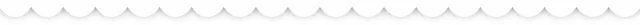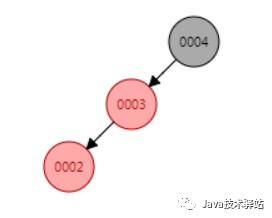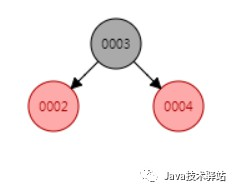# 【死磕 Java 集合】— TreeMap原始碼分析（二）### 插入元素

1. `public V put(K key, V value) {`
2. ` Entry t = root;`
3. ` if (t == null) {`
4. ` // 如果沒有根節點，直接插入到根節點`
5. ` compare(key, key); // type (and possibly null) check`
6. ` root = new Entry<>(key, value, null);`
7. ` size = 1;`
8. ` modCount++;`
9. ` return null;`
10. ` }`
11. ` // key比較的結果`
12. ` int cmp;`
13. ` // 用來尋找待插入節點的父節點`
14. ` Entry parent;`
15. ` // 根據是否有comparator使用不同的分支`
16. ` Comparator super K> cpr = comparator;`
17. ` if (cpr != null) {`
18. ` // 如果使用的是comparator方式，key值可以為null，只要在comparator.compare()中允許即可`
19. ` // 從根節點開始遍歷尋找`
20. ` do {`
21. ` parent = t;`
22. ` cmp = cpr.compare(key, t.key);`
23. ` if (cmp < 0)`
24. ` // 如果小於0從左子樹尋找`
25. ` t = t.left;`
26. ` else if (cmp > 0)`
27. ` // 如果大於0從右子樹尋找`
28. ` t = t.right;`
29. ` else`
30. ` // 如果等於0，說明插入的節點已經存在了，直接更換其value值並傳回舊值`
31. ` return t.setValue(value);`
32. ` } while (t != null);`
33. ` }`
34. ` else {`
35. ` // 如果使用的是Comparable方式，key不能為null`
36. ` if (key == null)`
37. ` throw new NullPointerException();`
38. ` @SuppressWarnings("unchecked")`
39. ` Comparable super K> k = (Comparable super K>) key;`
40. ` // 從根節點開始遍歷尋找`
41. ` do {`
42. ` parent = t;`
43. ` cmp = k.compareTo(t.key);`
44. ` if (cmp < 0)`
45. ` // 如果小於0從左子樹尋找`
46. ` t = t.left;`
47. ` else if (cmp > 0)`
48. ` // 如果大於0從右子樹尋找`
49. ` t = t.right;`
50. ` else`
51. ` // 如果等於0，說明插入的節點已經存在了，直接更換其value值並傳回舊值`
52. ` return t.setValue(value);`
53. ` } while (t != null);`
54. ` }`
55. ` // 如果沒找到，那麼新建一個節點，並插入到樹中`
56. ` Entry e = new Entry<>(key, value, parent);`
57. ` if (cmp < 0)`
58. ` // 如果小於0插入到左子節點`
59. ` parent.left = e;`
60. ` else`
61. ` // 如果大於0插入到右子節點`
62. ` parent.right = e;`
63.
64. ` // 插入之後的平衡`
65. ` fixAfterInsertion(e);`
66. ` // 元素個數加1（不需要擴容）`
67. ` size++;`
68. ` // 修改次數加1`
69. ` modCount++;`
70. ` // 如果插入了新節點傳回空`
71. ` return null;`
72. `}`

### 插入再平衡

1.

插入的元素如果是根節點，則直接塗成黑色即可，不用平衡；

2.

插入的元素的父節點如果為黑色，不需要平衡；

3.

插入的元素的父節點如果為紅色，則違背了特性4，需要平衡，平衡時又分成下麵三種情況：

（如果父節點是祖父節點的左節點）

1）父節點為紅色，叔叔節點也為紅色 （1）將父節點設為黑色；
（2）將叔叔節點設為黑色；
（3）將祖父節點設為紅色；
（4）將祖父節點設為新的當前節點，進入下一次迴圈判斷；
2）父節點為紅色，叔叔節點為黑色，且當前節點是其父節點的右節點 （1）將父節點作為新的當前節點；
（2）以新當節點為支點進行左旋，進入情況3）；
3）父節點為紅色，叔叔節點為黑色，且當前節點是其父節點的左節點 （1）將父節點設為黑色；
（2）將祖父節點設為紅色；
（3）以祖父節點為支點進行右旋，進入下一次迴圈判斷；

（如果父節點是祖父節點的右節點，則正好與上面反過來）

1）父節點為紅色，叔叔節點也為紅色 （1）將父節點設為黑色；
（2）將叔叔節點設為黑色；
（3）將祖父節點設為紅色；
（4）將祖父節點設為新的當前節點，進入下一次迴圈判斷；
2）父節點為紅色，叔叔節點為黑色，且當前節點是其父節點的左節點 （1）將父節點作為新的當前節點；
（2）以新當節點為支點進行右旋；
3）父節點為紅色，叔叔節點為黑色，且當前節點是其父節點的右節點 （1）將父節點設為黑色；
（2）將祖父節點設為紅色；
（3）以祖父節點為支點進行左旋，進入下一次迴圈判斷；

1. `/**`
2. ` * 插入再平衡`
3. ` *（1）每個節點或者是黑色，或者是紅色。`
4. ` *（2）根節點是黑色。`
5. ` *（3）每個葉子節點（NIL）是黑色。（註意：這裡葉子節點，是指為空(NIL或NULL)的葉子節點！）`
6. ` *（4）如果一個節點是紅色的，則它的子節點必須是黑色的。`
7. ` *（5）從一個節點到該節點的子孫節點的所有路徑上包含相同數目的黑節點。`
8. ` */`
9. `private void fixAfterInsertion(Entry x) {`
10. ` // 插入的節點為紅節點，x為當前節點`
11. ` x.color = RED;`
12.
13. ` // 只有當插入節點不是根節點且其父節點為紅色時才需要平衡（違背了特性4）`
14. ` while (x != null && x != root && x.parent.color == RED) {`
15. ` if (parentOf(x) == leftOf(parentOf(parentOf(x)))) {`
16. ` // a）如果父節點是祖父節點的左節點`
17. ` // y為叔叔節點`
18. ` Entry y = rightOf(parentOf(parentOf(x)));`
19. ` if (colorOf(y) == RED) {`
20. ` // 情況1）如果叔叔節點為紅色`
21. ` // （1）將父節點設為黑色`
22. ` setColor(parentOf(x), BLACK);`
23. ` // （2）將叔叔節點設為黑色`
24. ` setColor(y, BLACK);`
25. ` // （3）將祖父節點設為紅色`
26. ` setColor(parentOf(parentOf(x)), RED);`
27. ` // （4）將祖父節點設為新的當前節點`
28. ` x = parentOf(parentOf(x));`
29. ` } else {`
30. ` // 如果叔叔節點為黑色`
31. ` // 情況2）如果當前節點為其父節點的右節點`
32. ` if (x == rightOf(parentOf(x))) {`
33. ` // （1）將父節點設為當前節點`
34. ` x = parentOf(x);`
35. ` // （2）以新當前節點左旋`
36. ` rotateLeft(x);`
37. ` }`
38. ` // 情況3）如果當前節點為其父節點的左節點（如果是情況2）則左旋之後新當前節點正好為其父節點的左節點了）`
39. ` // （1）將父節點設為黑色`
40. ` setColor(parentOf(x), BLACK);`
41. ` // （2）將祖父節點設為紅色`
42. ` setColor(parentOf(parentOf(x)), RED);`
43. ` // （3）以祖父節點為支點進行右旋`
44. ` rotateRight(parentOf(parentOf(x)));`
45. ` }`
46. ` } else {`
47. ` // b）如果父節點是祖父節點的右節點`
48. ` // y是叔叔節點`
49. ` Entry y = leftOf(parentOf(parentOf(x)));`
50. ` if (colorOf(y) == RED) {`
51. ` // 情況1）如果叔叔節點為紅色`
52. ` // （1）將父節點設為黑色`
53. ` setColor(parentOf(x), BLACK);`
54. ` // （2）將叔叔節點設為黑色`
55. ` setColor(y, BLACK);`
56. ` // （3）將祖父節點設為紅色`
57. ` setColor(parentOf(parentOf(x)), RED);`
58. ` // （4）將祖父節點設為新的當前節點`
59. ` x = parentOf(parentOf(x));`
60. ` } else {`
61. ` // 如果叔叔節點為黑色`
62. ` // 情況2）如果當前節點為其父節點的左節點`
63. ` if (x == leftOf(parentOf(x))) {`
64. ` // （1）將父節點設為當前節點`
65. ` x = parentOf(x);`
66. ` // （2）以新當前節點右旋`
67. ` rotateRight(x);`
68. ` }`
69. ` // 情況3）如果當前節點為其父節點的右節點（如果是情況2）則右旋之後新當前節點正好為其父節點的右節點了）`
70. ` // （1）將父節點設為黑色`
71. ` setColor(parentOf(x), BLACK);`
72. ` // （2）將祖父節點設為紅色`
73. ` setColor(parentOf(parentOf(x)), RED);`
74. ` // （3）以祖父節點為支點進行左旋`
75. ` rotateLeft(parentOf(parentOf(x)));`
76. ` }`
77. ` }`
78. ` }`
79. ` // 平衡完成後將根節點設為黑色`
80. ` root.color = BLACK;`
81. `}`

### 插入元素舉例

（1）將父節點作為新的當前節點；

（2）以新當節點為支點進行左旋，進入情況3）；（1）將父節點設為黑色；

（2）將祖父節點設為紅色；

（3）以祖父節點為支點進行右旋，進入下一次迴圈判斷；